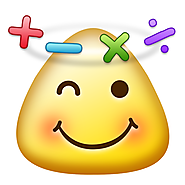Updated by Amanda Hale on Sep 02, 2015
REPORTAmanda Hale
Owner
10 items   2 followers   0 votes   162 views

# iPad Apps for MS Fractions, Decimals, and Percents Class

1

## Virtual Manipulatives!*FREE*
I have provided a virtual set of fraction bars on www.abcya.com for several years. Many teachers have contacted me about creating a similar application for the iPad. Here it is! I offer this app for free because virtual manipulatives alone do not provide meaning for learners; they require a teacher to guide meaning and connections.

2

## Fractions - by Brainingcamp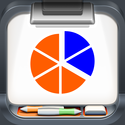*FREE*
"The most comprehensive fractions app I've seen. This app should be in every classroom!" -- BestAppsForKids.org
"If you have a student or child that has difficulty with fractions then this is the app for you." -- SmartAppsForKids.com

Everything you need for teaching and learning: (1) Narrated lesson (2) Practice questions (3) Virtual manipulative (4) Challenging game

3

## Oh No Fractions! - Curious Hat Lab*FREE version* (Full version is \$0.99)
Practice and explore fractions with this elegant and simple visual app.

Swipe left or right to answer if the fraction on the left is LESS, GREATER or EQUAL than the one on the right.
Peek at the visual solution before committing your answer or wait until later to prove it.
Tap above each fraction to see if they can be reduced/simplified.

5

## Fraction Basics*FREE*
This free video app provides a quick and simple way for you to learn and understand the basics behind fractions. There are 12 computer animated videos on understanding fractions, improper fractions & mixed fractions, equivalent fractions, adding fractions, adding fractions examples, subtracting fractions, multiplying fractions, multiplying fractions examples, dividing fractions, dividing fractions examples, convert fraction to percent, convert decimal to fraction. Each of these videos is divided into several different parts that you can easily skipped to.

6

## Math Practice for Fraction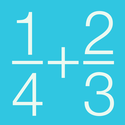*FREE*
PRACTICE IS THE KEY TO BE GOOD AT MATH
GENERATE A LOT OF MATH PROBLEMS TO PRACTICE

The way to be good at math is to solve a lot of problems with step-by-step.
Math Practice gives you a lot of math problems.
It generates problems of four operations. +, -, ×, ÷

7

## DigitWhiz Math*FREE*
Master foundational math skills in a FUN way! DigitWhiz has helped thousands of kids ages 8+ master basic and pre-algebra skills.

8*FREE*
How good is your mental math?

The 24 Game is a math puzzle game for all ages who wants to exercise or improve their quick thinking and mental math.

The goal of the game is to combine 4 numbers into 1 number, equal to 24 by adding, subtracting, and multiplying.

9

## Long Division Touch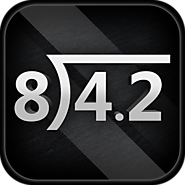*FREE*

Learn the mechanics of long division with a touch interface. Drag digits down, slide the decimal into the correct position, and tap to identify repeating decimals.

Say you have 8)256. Drag 8 into ‘25’ and find the correct multiple. Then drag the 6 down and finish the problem!

Learning long division can be enjoyable when you’re not slowed down by pencil/paper or lost without instant feedback.

Master long division by practicing on your iPhone, iPad or iPod Touch!

Lessons include:
Long Division Basics

Zero up top
Remainders
Decimals
Repeating Decimals
Decimals in the Divisor

Random problem generators for each lesson are available with an in-app purchase.

10

## Math Cards ! !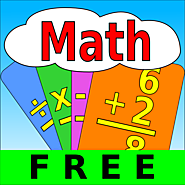*FREE*

Math Cards ! ! is a great way to learn and practice addition, subtraction, multiplication, and division. Customize your own problem sets to focus on your needs. Set the top numbers and bottom numbers for each operator.

Lite Version Features:
1) Choose from the following operators: +, -, x, ÷
3) Set the top and bottom numbers for subtraction
4) Set the top and bottom factors for multiplication
5) Set the divisors and quotients for division
6) Allow negative answers for subtraction if desired
7) Set the timer on or off
8) Set the number of problems in the problem set

If you like this app, please consider giving it a rating. Your support will help keep the updates coming! Thank you for your support!

11